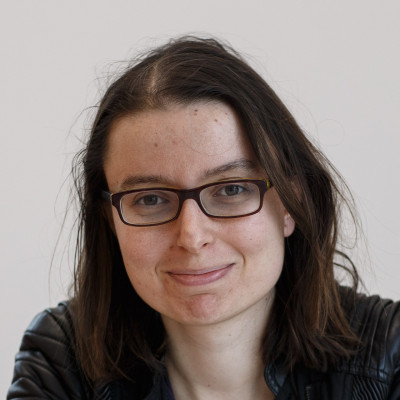# Dr Clelia Pech

Lecturer in Mathematics

`Clélia joined SMSAS in summer 2015 from a postdoctoral research position at the Institut Camille Jordan in Lyon having previously lectured in Geometry at King's College London. Her PhD, supervised by Laurent Manivel at the Institut Fourier, Grenoble dealt with the quantum cohomology of odd symplectic Grassmannians. She is a member of the School’s Placements team. `

## Research interests

`Homogeneous and quasi-homogeneous varietiesMirror symmetryQuantum cohomologyDerived categoriesSchubert calculus `

## Supervision

`Clélia is supervising research student Peter Spacek (Mirror symmetry for exceptional homogeneous spaces)`

## Professional

`Clélia is the School’s representative to the London Mathematical Society and she is also an organiser of the COW Seminar. This is an algebraic geometry seminar meeting in various locations across the southern United Kingdom.`

## Publications

### Article

• Langlois, K., Pech, C. and Raibaut, M. (2019). Stringy invariants for horospherical varieties of complexity one. Algebraic Geometry [Online] 6:346-383. Available at: http://dx.doi.org/10.14231/AG-2019-017.
We determine the stringy motivic volume of log terminal horospherical varieties of complexity one and obtain a smoothness criterion using a comparison of stringy and usual Euler characteristics.
• Pech, C. and Rietsch, K. (2018). A comparison of Landau-Ginzburg models for odd-dimensional Quadrics. Bulletin of the Institute of Mathematics Academia Sinica [Online] 13:249-291. Available at: https://doi.org/10.21915/BIMAS.2018301.
In [Rie08], the second author defined a Landau-Ginzburg model for homogeneous spaces G/P, as a regular function on an affine subvariety of the Langlands dual group. In this paper, we reformulate this LG model (X^, W_t) in the case of the odd-dimensional quadric, as a rational function on a Langlands dual projective space, in the spirit of work by R. Marsh and the second author for type A Grassmannians and by both authors for Lagrangian Grassmannians. We also compare this LG model with the one obtained independently by Gorbounov and Smirnov, and we use this comparison to deduce part of a conjecture of the second author for odd-dimensional quadrics.
• Pech, C., Rietsch, K. and Williams, L. (2016). On Landau-Ginzburg models for quadrics and flat sections of Dubrovin connections. Advances in Mathematics [Online] 300:275-319. Available at: http://dx.doi.org/10.1016/j.aim.2016.03.020.
This paper proves a version of mirror symmetry expressing the (small) Dubrovin connection for even-dimensional quadrics in terms of a mirror-dual Landau–Ginzburg model View the MathML source(X?can,Wq). Here X?can is the complement of an anticanonical divisor in a Langlands dual quadric. The superpotential Wq is a regular function on X?can and is written in terms of coordinates which are naturally identified with a cohomology basis of the original quadric. This superpotential is shown to extend the earlier Landau–Ginzburg model of Givental, and to be isomorphic to the Lie-theoretic mirror introduced in . We also introduce a Laurent polynomial superpotential which is the restriction of Wq to a particular torus in X?can. Together with results from  for odd quadrics, we obtain a combinatorial model for the Laurent polynomial superpotential in terms of a quiver, in the vein of those introduced in the 1990's by Givental for type A full flag varieties. These Laurent polynomial superpotentials form a single series, despite the fact that our mirrors of even quadrics are defined on dual quadrics, while the mirror to an odd quadric is naturally defined on a projective space. Finally, we express flat sections of the (dual) Dubrovin connection in a natural way in terms of oscillating integrals associated to View the MathML source(X?can,Wq) and compute explicitly a particular flat section.
• Pech, C. (2014). Quantum Product and Parabolic Orbits in Homogeneous Spaces. Quantum Product and Parabolic Orbits in Homogeneous Spaces [Online] 42:4679-4695. Available at: http://www.dx.doi.org/10.1080/00927872.2013.820736.
Chaput, Manivel, and Perrin proved in  a formula describing the quantum product by Schubert classes associated to cominuscule weights in a rational projective
homogeneous space X. In the case where X has Picard rank one, we relate this formula to the stratification of X by P-orbits, where P is the parabolic subgroup associated to the cominuscule weight. We deduce a decomposition of the Hasse diagram of X, i.e., the diagram describing the cup-product with the hyperplane class. For all classical Grassmannians, we give a complete description of parabolic orbits associated to cominuscule weights, and we make the decomposition of the Hasse diagram explicit.

### Thesis

• Nolan, B. (2017). A Strong Dixmier-Moeglin Equivalence for Quantum Schubert Cells and an Open Problem for Quantum Plücker Coordinates.
In this thesis, the algebras of primary interest are the quantum Schubert cells and the quantum Grassmannians, both of which are known to satisfy a condition on primitive ideals known as the Dixmier-Moeglin equivalence.
A stronger version of the Dixmier-Moeglin equivalence is introduced - a version which deals with all prime ideals of an algebra rather than just the primitive ideals. Quantum Schubert cells are shown to satisfy the strong Dixmier-Moeglin equivalence.
Until now, given a torus-invariant prime ideal of the quantum Grassmannian, one
could not decide which quantum Plücker coordinates it contains. Presented here is a graph-theoretic method for answering this question. This may be useful for providing a full description of the inclusions between the torus-invariant prime ideals of the quantum Grassmannian and may lead to a proof that quantum Grassmannians satisfy the strong Dixmier-Moeglin equivalence.

### Forthcoming

• Lee, C. (2019). On the Invariant Rings of Modular Bireflection Groups With Applications of Macaulay’s Double Annihilator Correspondence.
Let V be a faithful finite-dimensional representation of a finite group G over an odd prime field k, and S = k[V], the symmetric algebra on the dual V*. Chapter 2 shows how to find the invariant ring S^G when G is an abelian unipotent two-row group. The invariant rings are complete intersections.
Chapter 3 shows an algorithm that computes the Macaulay inverse for any homogenous (S^+)-primary irreducible ideal of S. It will also be shown that the Hilbert ideal of the invariant rings of the abelian two-row groups from chapter 2 are complete intersection ideals with inverse monomials as Macaulay inverses.
Last updated